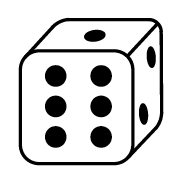### Home > CC2MN > Chapter 7 > Lesson 7.1.1 > Problem7-9

7-9.Desmond is rolling a standard six-sided number cube. He plans to roll it $72$ times.

1. About how many times would you expect Desmond to roll a $4$? Why?

You would expect Desmond to roll a $4$ about one sixth of the total rolls, $72$, since there are $6$ numbers on a cube. What is one sixth of $72$?

2. About how many times would you expect him to roll an even number? Why?

See part (a).

There are three even numbers on a cube.

1. Desmond kept track of his results for all $72$ rolls. The table at right shows some of his results. Based on his partial results, how many times did he roll a $5$ or a $6$?

What is the total Number of Outcomes in the table on the right? How many more times did Desmond roll the cube?

Result

Number of Outcomes

1

9

2

14

3

11

4

8

Use the eTool below to solve problem.
Click the link at right for the full version of the eTool: 7-9 HW eTool